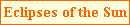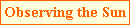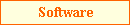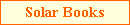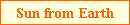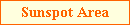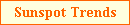One advantage of making disk drawings is that the area of sunspots can be measured. The units of sunspot area is millionths of the Sun's visible hemisphere. From each daily observation, the total sunspot area can be measured and a monthly average calculated. This is shown below with area in millionths of the Sun's visisble hemipshere.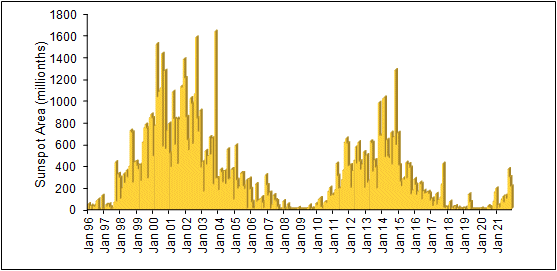The maximum area of individual groups as they progress across the solar disk can also be determined. This is shown below, again with area in millionths of the Sun's visible hemipshere (yellow for groups smaller than 100 millionths, green for groups between 100 millionths and 500 millionths, purple for groups between 500 millionths and 1000 millionths and red for groups greater than 1000 millionths). If the area of a group is very small (such as for A and B type groups) then it is given an area of zero. The largest number of high area groups usually occur one to two years before and after sunspot maximum. For comparison, the largest sunspot group on record had an area of 6100 millionths on 8th April 1947.Three methods to determine sunspot area are described below:-

The most accurate method to calculate sunspot area is through the creation of a pixel mask (i.e. for all the pixels containing a sunspot) followed by the calculation of each the foreshortened area of pixel in the mask.  By simplying adding the area of each pixel gives the area of either all sunspot groups on the disk or of individual groups.  The mask can be created using the freeware Windows Helio Image Creator program followed by manually ensuring that all pixels of the sunspots are part of the mask and using Helio Viewer to calculate the area.  See the software page for further details of the software and their user guides. An example drawings together with a mask for the large sunspot group AR 12192 is shown below.An example of using this mask method of calculating sunspot area can be found in a paper 'Remeasurement of Solar Observing Optical Network sunspot areas' available at https://doi.org/10.1093/mnras/staa2007.

Grid Method

The second method using a square grid and the Windoes Helio or HelioViewer software, to easily calculate sunspot area. The only inputs are the date/time, the position of the sunspot (x and y coordinates with respect to the centre of the disk) and number of grid squares covering the sunspot.  Grid of either 1mm by 1mm or 1/25in by 1/25in can be used (these are provided below).

Mathematical and Graphical Method

The final method is to manually calculate the area of a group using an equation and the same grid as in the second example above. The expression to calculate the area of a sunspot group (corrected for foreshortening) is:-where:-

• AM = sunspot area in millionths of the Sun's visible hemisphere,
•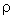= the angular distance on the surface of the Sun from the centre of the disk to the group,
• AS = measured sunspot area (e.g. square mm or square inch),
• R = radius of solar drawing (e.g. mm or inches),
• B = heliographic latitude of sunspot group (degrees),
• L = heliographic longitude of sunspot group (degrees) and
• L0 = heliographic longitude of the centre of the disk (degrees).

This equation simplifies to the following for given disk diameters and grid sizes:-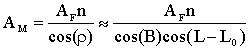where:-

• AF = area factor that is dependant on disk diameter and grid size (see table below) and
• n = the number of grid squares covering the penumbral and umbral regions of all the spots in a group.
 Disk Diameter Grid Size (PDF) Grid Size (GIF) AF 100 mm 63.66 125 mm 40.74 150 mm 28.29 4 inch 63.66 5 inch 40.74 6 inch 28.29To the left is a half size angular distance chart showingat intervals of 10°. Placing the chart of the appropriate diameter over the drawing disk enables the angular diameter angleto be determined and used in the above equations. The following table gives a set of charts:-

 PDF 100 mm 125 mm 150mm 4 in 5 in 6 in GIF 100 mm 125 mm 150 mm 4 in 5 in 6 in

For example, for a disk drawing of 150 mm diameter with all the spots in a group covering ten 1 mm by 1 mm grid squares (i.e. AF=28.29 and n=10) and= 27.5° the corrected area of the group would be 320 millionths of the Sun's visible hemipshere.Last updated on 26 September 2020.